# Calculate Angles

In this worksheet, students will be asked to calculate the missing angles on a straight line, in a quadrilateral, around a point and on an intersecting line.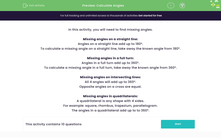Key stage:  KS 2

Curriculum topic:   Geometry: Properties of Shapes

Curriculum subtopic:   Recognise/Find Angles

Popular topics:   Geometry worksheets

Difficulty level:#### Worksheet Overview

In this activity, you will need to find missing angles.

Missing angles on a straight line:

Angles on a straight line add up to 180°.

To calculate a missing angle on a straight line, take away the known angle from 180°.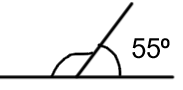180° - 55° = 125°

Missing angles in a full turn:

Angles in a full turn add up to 360°.

To calculate a missing angle in a full turn, take away the known angle from 360°.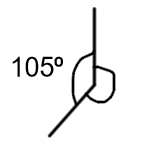360° - 105° = 255°

Missing angles on intersecting lines:

All 4 angles will add up to 360°.

Opposite angles on a cross are equal.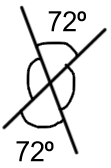360° - 72° - 72° = 216°

216° ÷ 2 = 108°

Both missing angles are 108°

A quadrilateral is any shape with 4 sides.

For example: square, rhombus, trapezium, parallelogram.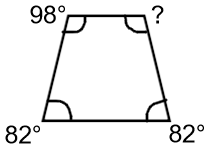360° - 82° - 82° - 98° = 98°

We can also see that, in this trapezium, opposite angles are the same.

### What is EdPlace?

We're your National Curriculum aligned online education content provider helping each child succeed in English, maths and science from year 1 to GCSE. With an EdPlace account you’ll be able to track and measure progress, helping each child achieve their best. We build confidence and attainment by personalising each child’s learning at a level that suits them.

Get started